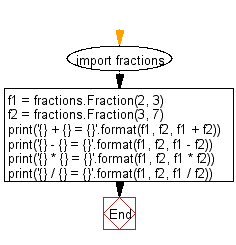﻿ Python Math: Add, subtract, multiply and divide two fractions - w3resource# Python Math: Add, subtract, multiply and divide two fractions

## Python Math: Exercise-46 with Solution

Write a Python program to add, subtract, multiply and divide two fractions.

Sample Solution:-

Python Code:

``````import fractions

f1 = fractions.Fraction(2, 3)
f2 = fractions.Fraction(3, 7)

print('{} + {} = {}'.format(f1, f2, f1 + f2))
print('{} - {} = {}'.format(f1, f2, f1 - f2))
print('{} * {} = {}'.format(f1, f2, f1 * f2))
print('{} / {} = {}'.format(f1, f2, f1 / f2))
```
```

Sample Output:

```2/3 + 3/7 = 23/21
2/3 - 3/7 = 5/21
2/3 * 3/7 = 2/7
2/3 / 3/7 = 14/9
```

Flowchart:Python Code Editor:

Have another way to solve this solution? Contribute your code (and comments) through Disqus.

What is the difficulty level of this exercise?

Test your Programming skills with w3resource's quiz.

﻿

```>>> students = [{'name': 'John', 'score': 98}, {'name': 'Mike', 'score': 94}, {'name': 'Jennifer', 'score': 99}]